# Non Verbal Reasoning - Series

### Exercise :: Series - Section 1

Each of the following questions consists of five figures marked A, B, C, D and E called the Problem Figures followed by five other figures marked 1, 2, 3, 4 and 5 called the Answer Figures. Select a figure from amongst the Answer Figures which will continue the same series as established by the five Problem Figures.

16.

Select a figure from amongst the Answer Figures which will continue the same series as established by the five Problem Figures.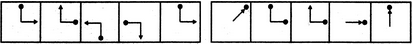(A)     (B)     (C)     (D)     (E)       (1)     (2)     (3)     (4)     (5)

 A. 1 B. 2 C. 3 D. 4 E. 5

Explanation:

In each step, the pin rotates 90oCW and the arrow rotates 90oACW.

17.

Select a figure from amongst the Answer Figures which will continue the same series as established by the five Problem Figures.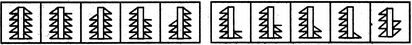(A)     (B)     (C)     (D)     (E)       (1)     (2)     (3)     (4)     (5)

 A. 1 B. 2 C. 3 D. 4 E. 5

Explanation:

In one step, the figure gets laterally inverted and one line segment is lost from the upper end of the RHS portion of the figure. In the next step, the figure gets laterally inverted and one line segment is lost from the upper end of the LHS portion of the figure.

18.

Select a figure from amongst the Answer Figures which will continue the same series as established by the five Problem Figures.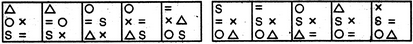(A)     (B)     (C)     (D)     (E)       (1)     (2)     (3)     (4)     (5)

 A. 1 B. 2 C. 3 D. 4 E. 5

Explanation:

The elements move in the sequence's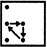andalternately.

19.

Select a figure from amongst the Answer Figures which will continue the same series as established by the five Problem Figures.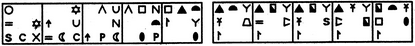(A)     (B)     (C)     (D)     (E)       (1)     (2)     (3)     (4)     (5)

 A. 1 B. 2 C. 3 D. 4 E. 5

Explanation:

All the elements move half-a-side of the square boundary in ACW direction in each step. Also, first, third and fifth elements are replaced by new elements in one step and second, fourth and sixth elements are replaced by new elements in the next step. The two steps are repeated alternately.

20.

Select a figure from amongst the Answer Figures which will continue the same series as established by the five Problem Figures.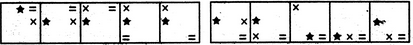(A)     (B)     (C)     (D)     (E)       (1)     (2)     (3)     (4)     (5)

 A. 1 B. 2 C. 3 D. 4 E. 5